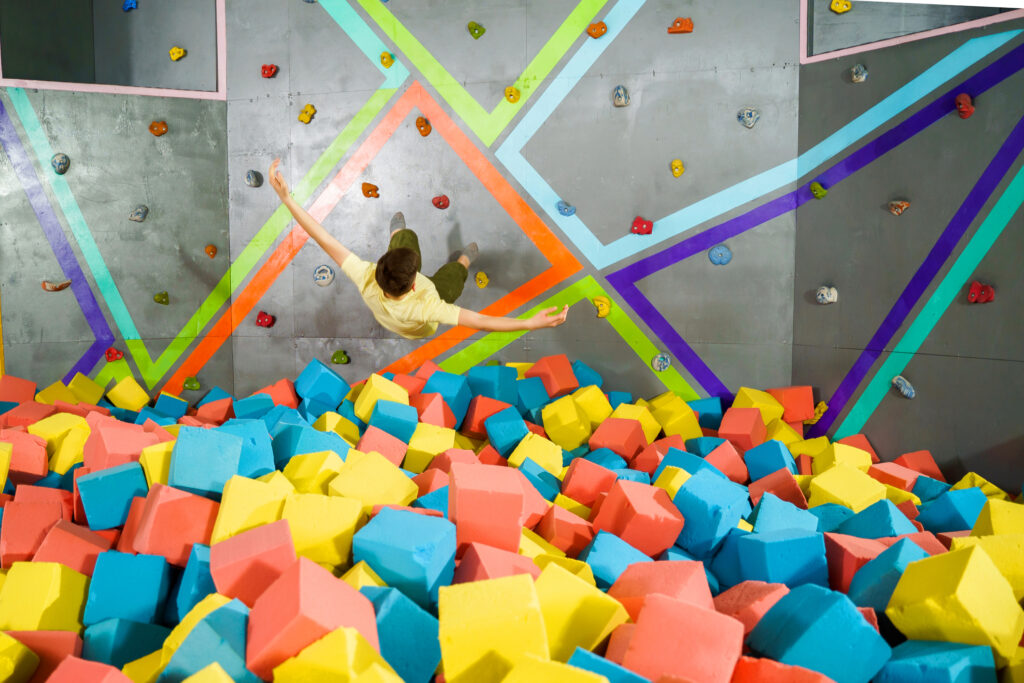# Foam Pit Block Calculator

#### Calculate how many foam cubes you need in order to fill a gymnastics pit.## Use our foam put block calculator to find out how many 6 or 8 inch cubes you're going to need!

If you know the dimensions of your pit, and whether you will need 6″ or 8″ cubes to fill your gymnastics pit, then you can use our calculator below to determine exactly how many cubes you will need to fill it to the top. Contact us if you have an L-shaped pit and need to determine how many blocks will fit inside.

Length*
Length of the pit (in inches).
Width*
Width of the pit (in inches).
Depth*
Depth of the pit (in inches).
Total 6" Blocks:
Blocks
Here's what you will need:
Total 8" Blocks:
Blocks
Here's what you will need:
Name*
Contact person name
Email*